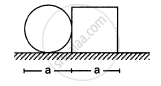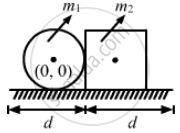Department of Pre-University Education, KarnatakaPUC Karnataka Science Class 11
Advertisement Remove all ads

# A Circular Plate of Diameter D is Kept in Contact with a Square Plate of Edge D as Show in Figure. the Density of the Material and the Thickness Are Same Everywhere. - Physics

MCQ

A circular plate of diameter d is kept in contact with a square plate of edge d as show in figure. The density of the material and the thickness are same everywhere. The centre of mass of the composite system will be#### Options

• inside the circular plate

• inside the square plate

• at the point of contact

• outside the system.

Advertisement Remove all ads

#### Solution

inside the square plate

Let m1 be the mass of circular plate and m2 be the mass of square plate.
The thickness of both the plates is t.
$\text{ mass = density × volume}$
$m_1 = \rho\pi \left( \frac{d}{2} \right)^2 t$
$m_2 = \rho d^2 t$

Centre of mass of the circular plate lies at its centre.
Let the centre of circular plate be the origin.
$\vec{r}_1 = 0$
Centre of mass of the square plate lies at its centre.
$\vec{r}_2 = 2d$
$\text{Now,}$
$R = \frac{m_1 \vec{r}_1 + m_2 \vec{r}_2}{m_1 + m_2}$
$= \frac{m_1 \times 0 + \rho d^2 t \times 2d}{\rho\pi \left( \frac{d}{2} \right)^2 t + \rho d^2 t}$
$= \frac{2d}{\frac{\pi}{4} + 1} = 1 . 12d$
$\Rightarrow \text{R > d}$
$\therefore$ Centre of mass of the system lies in the square plate.Is there an error in this question or solution?
Advertisement Remove all ads

#### APPEARS IN

HC Verma Class 11, 12 Concepts of Physics 1
Chapter 9 Centre of Mass, Linear Momentum, Collision
MCQ | Q 6 | Page 157
Advertisement Remove all ads
Advertisement Remove all ads
Share
Notifications

View all notifications

Forgot password?